## B.5A Multi-Antenna channel models using cross polarized antennas

36.1413GPPBase Station (BS) conformance testingEvolved Universal Terrestrial Radio Access (E-UTRA)Release 17TS

The MIMO channel correlation matrices defined in B.5A apply to two cases as presented below:

– One TX antenna and multiple RX antennas case, with cross polarized antennas used at eNodeB;

– Multiple TX antennas and multiple RX antennas case, with cross polarized antennas used at both UE and eNodeB.

The cross-polarized antenna elements with +/-45 degrees polarization slant angles are deployed at eNB. For one TX antenna case, antenna element with +90 degree polarization slant angle is deployed at UE. For multiple TX antennas case, cross-polarized antenna elements with +90/0 degrees polarization slant angles are deployed at UE.

For the cross-polarized antennas, the N antennas are labelled such that antennas for one polarization are listed from 1 to N/2 and antennas for the other polarization are listed from N/2+1 to N, where N is the number of TX or RX antennas.

## B.5A.1 Definition of MIMO Correlation Matrices using cross polarized antennas

For the channel spatial correlation matrix, the following is used: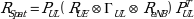Where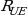is the spatial correlation matrix at the UE with same polarization,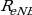is the spatial correlation matrix at the eNB with same polarization,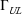is a polarization correlation matrix,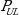is a permutation matrix, and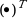denotes transpose.

Table B.5A.1-1 defines the polarization correlation matrix.

Table B.5A.1-1: Polarization correlation matrix

 One TX antenna Multiple TX antennas Polarization correlation matrix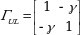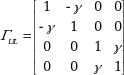The matrix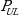is defined as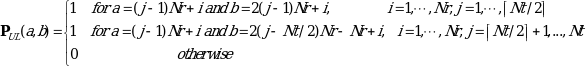where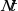and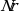is the number of TX and RX antennas respectively, and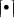is the ceiling operator.

The matrix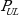is used to map the spatial correlation coefficients in accordance with the antenna element labelling system described in B.5A.

## B.5A.2 Spatial Correlation Matrices at UE and eNB sides

### B.5A.2.1 Spatial Correlation Matrices at UE side

For 1-antenna transmitter,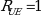.

For 2-antenna transmitter using one pair of cross-polarized antenna elements,.

For 4-antenna transmitter using two pairs of cross-polarized antenna elements,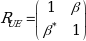.

### B.5A.2.2 Spatial Correlation Matrices at eNB side

For 2-antenna receiver using one pair of cross-polarized antenna elements,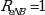.

For 4-antenna receiver using two pairs of cross-polarized antenna elements,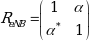.

For 8-antenna receiver using four pairs of cross-polarized antenna elements,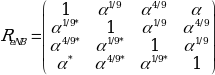.

## B.5A.3 MIMO Correlation Matrices using cross polarized antennas

The values for parameters α, β and γ for low spatial correlation are given in Table B.5A.3-1.

Table B.5A.3-1: Values for parameters α,  and γ

 Low spatial correlation    0 0 0 Note 1: Value of α applies when more than one pair of cross-polarized antenna elements at eNB side. Note 2: Value of β applies when more than one pair of cross-polarized antenna elements at UE side.

The correlation matrices for low spatial correlation are defined in Table B.5A.3-2 as below.

Table B.5A.3-2: MIMO correlation matrices for low spatial correlation

 1×8 case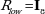2×8 case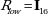In Table B.5A.3-2,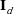is a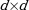identity matrix.